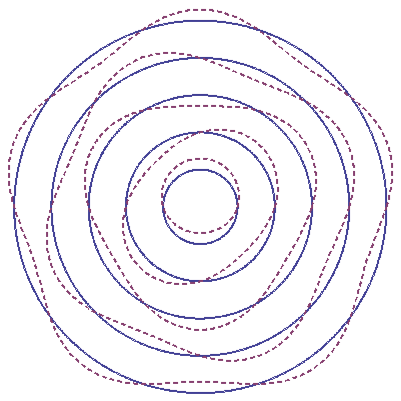## Section54.1Matter Waves

Louis De Broglie in France had a breakthrough in thinking when he asked: if a photon can be have both particle and wave properties, why can't an electron also have particle and wave properties? He thought that, if electrons were waves, one could understand the stability of electronic orbits, called Bohr orbits (to be addressed in the next chapter), similar to the stability of standing waves on a string. For instance, the size of a Bohr orbit could correspond to an integral multiple of the wavelength of the electron wave as illustrated in Figure 54.1.1.Figure 54.1.1. The figure shows de Broglie wave pictures for several Bohr orbits. The picture was generated using the program at Bohr's Orbits 1 .
demonstrations.wolfram.com

Thus, for a Bohr orbit of radius $r\text{,}$ there will be a natural quantization:

\begin{equation} 2\pi r = n \lambda,\tag{54.1.1} \end{equation}

where $\lambda$ would be the wavelength of the electron wave and $n$ some integer. But, what should be the wavelength of an electron? He used the analogy with photon and proposed a parallel concept for electrons as shown in Table 54.1.2.

With this assumption de Broglie could account for the discrete spectrum of Hydrogen atom quite naturally. With the success of thinking electrons as waves de Broglie stated that the wave picture should apply to all material particles.

The question that we can now ask is: Why do we not see wave-like properties of ordinary objects? To that question, de Broglie's answer is that their wavelengths are too small compared to the dimensions of the object. Therefore, even though they are waves, we cannot see their wave aspects so easily. In the following we will work out some examples of de Broglie wavelengths.

What is the de Broglie wavelength of an electron moving at $1.0\times 10^{5}\:\textrm{m/s}\text{?}$

Hint

Use momentum.

$7.3\:\textrm{nm}\text{.}$

Solution

First we need to decide if we will use the relativistic formula or the non-relativistic formula for calculating the momentum. Here we have $v/c = 0.33\times 10^{-3}\text{,}$ which is much very small compared to $1\text{.}$ Therefore, we will use the non-relativistic formula.

\begin{equation*} p = m v = 9.1\times 10^{-31}\:\textrm{kg} \times 1.0\times 10^{5}\:\textrm{m/s} = 9.1\times 10^{-26}\:\textrm{kg.m/s}. \end{equation*}

Therefore, the wavelength of the electron will be

\begin{equation*} \lambda = \dfrac{h}{p} = \dfrac{6.63\times10^{-34}\textrm{J.s}}{9.1\times 10^{-26}\:\textrm{kg.m/s}} = 7.3\:\textrm{nm}. \end{equation*}

What is the de Broglie wavelength of a ball of mass $0.350\:\textrm{kg}$ moving at $45.0\:\textrm{m/s}\text{?}$

Hint

Use momentum.

$4.2\times 10^{-35}\:\textrm{m}\text{.}$

Solution

This is a non-relativistic situation.

\begin{equation*} p = m v = 0.350\:\textrm{kg} \times 45.0\:\textrm{m/s} = 15.75\:\textrm{kg.m/s}. \end{equation*}

Therefore, the wavelength of the electron will be

\begin{equation*} \lambda = \dfrac{h}{p} = \dfrac{6.63\times10^{-34}\textrm{J.s}}{15.75\:\textrm{kg.m/s}} = 4.2\times 10^{-35}\:\textrm{m}. \end{equation*}

Clearly, this is too small a wavelength for the wave picture to be of much use.

What is the de Broglie wavelength of an electron which has a kinetic energy of $100\:\textrm{keV}\text{?}$

Hint

Use relativistic energy formula.

$5.9\times 10^{-13}\:\textrm{m}\text{.}$

Solution

Rest energy of an electron is $511\text{ keV}\text{.}$ Since the kinetic energy is not too small compared to the rest energy, the particle should be treated relativistically.

\begin{equation*} K = E - mc^2 = \sqrt{p^2c^2 + m^2 c^4} - m c^2. \end{equation*}

Therefore, $pc$ will be

\begin{equation*} (pc)^2 = \left( K + m c^2 \right)^2 - (m c^2)^2 = K^2 + 2 K m c^2. \end{equation*}

Therefore, the wavelength will be

\begin{equation*} \lambda = \dfrac{hc}{pc} = \dfrac{hc}{\sqrt{K^2 + 2 K m c^2}}. \end{equation*}

Now, if we use the value of $hc$ in units $\textrm{eV.m}$ and the energies in $\textrm{eV}$ we will not have to change too many units.

\begin{equation*} hc = 6.58\times 10^{-16} \textrm{eV.s} \times 3.0\times 10^{8}\textrm{m/s} = 1.97\times 10^{-7}\:\textrm{eV.m}, \end{equation*}

and

\begin{align*} K^2 + 2 K m c^2 \amp = (10^5\:\textrm{eV})^2 + 2 \times 10^5\:\textrm{eV}\times 5.11 \times 10^5\:\textrm{eV}\\ \amp \approx 11.2 \times 10^{10}\:\textrm{eV}^2. \end{align*}

Therefore,

\begin{equation*} \lambda = \dfrac{1.97\times 10^{-7}\:\textrm{eV.m}}{\sqrt{11.2 \times 10^{10}\:\textrm{eV}^2}} = 5.9\times 10^{-13}\:\textrm{m}. \end{equation*}
TODO: \​begin{exercise} What is the wavelength of a 50-kg person running at 10 m/s? \end{exercise} \​begin{exercise} What is the wavelength of an electron ($m_e = 9.1 \times 10^{-31}$ kg) moving at (a) 10 m/s, (b) $10^4$ m/s, (c) $10^8$ m/s? \end{exercise} \​begin{exercise} What is the wavelength of a proton ($m = 1.67 \times 10^{-27}$ kg) moving at (a) 10 m/s, (b) $10^4$ m/s, (c) $10^8$ m/s? \end{exercise} \​begin{exercise} (a) What is the wavelength of a 100 keV photon? (b) What is the wavelength of a 100 keV electron? \end{exercise} \​begin{exercise} What is the wavelength of a 1 TeV proton in an accelerator? \end{exercise} \​begin{exercise} (a) At what energy would an electron have the wavelength 0.5 $\mu$m, which is the wavelength of a light in the visible spectrum? (b) How does this energy compare with the energy of a photon of wavelength 0.5 $\mu$m? \end{exercise} \​begin{exercise} (a) The momentum of a nonrelativistic particle is doubled, what becomes of its wavelength? (b) What will be the case if the particle was relativistic? \end{exercise} \​begin{exercise} (a) The kinetic energy of a nonrelativistic particle is doubled, what becomes of its wavelength? (b) What will be the case if the particle was relativistic? \end{exercise}